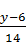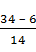### Sample Problem

Solve the equation 14x + 4 = y – 2 for x if y is 34. Make sure to first solve the equation for x in terms of y.

x =

#### Solution

14x = y - 6

x =,

If y = 34,

x =x == 2